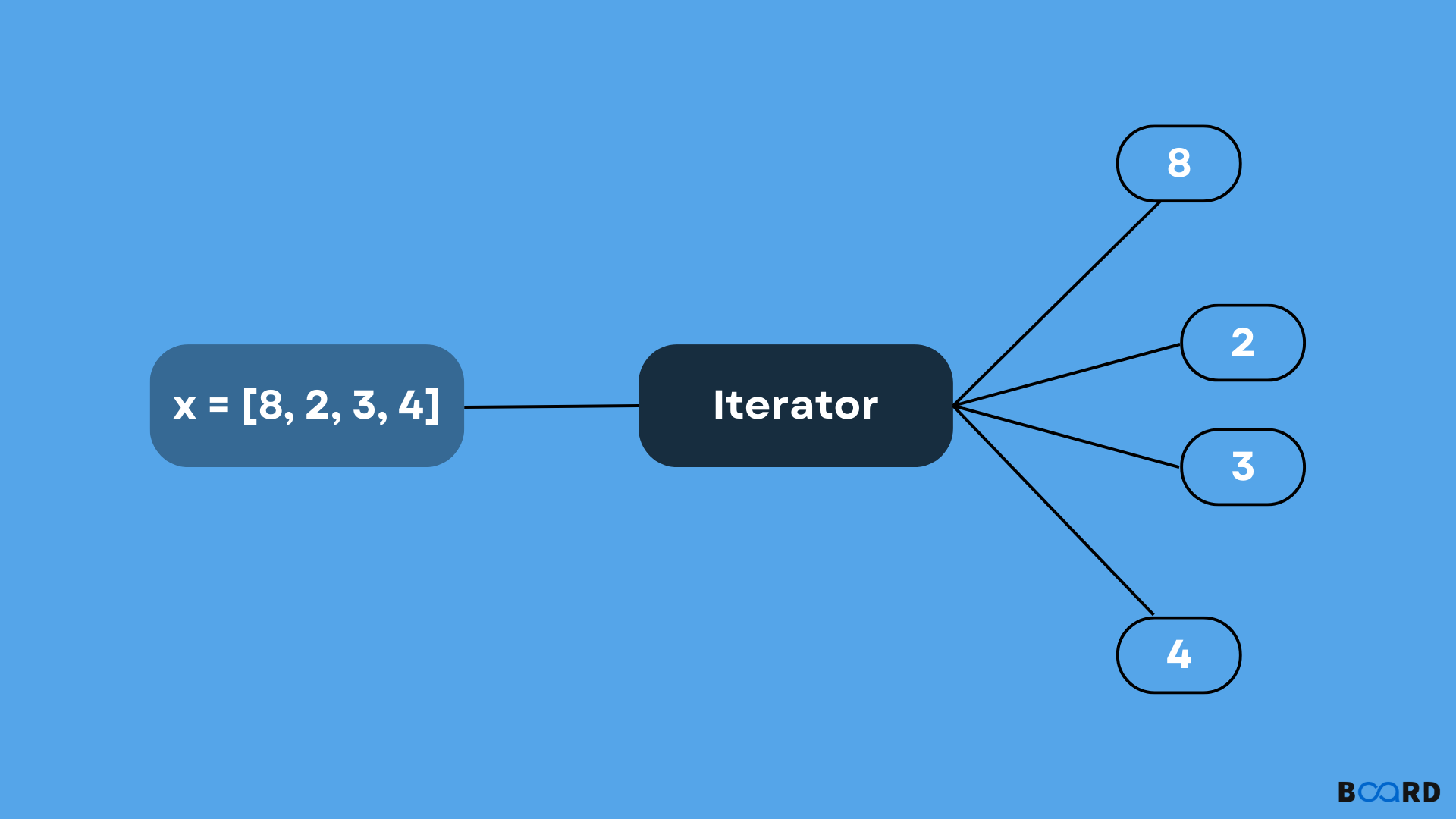# Iterators in PythonIn python, an object called an iterator is used to iterate over iterable objects such as lists, tuples, dictionaries etc. To initialize this object we need to use the iter() method. For iteration, this object uses the next() method.

## iter() method

This method is used for initializing the iterator. This method returns an iterator object.

## next() method

For getting the next value of the iterator we use the next() method. While using a loop over an iterable object, it makes use of the iter() object to get an iterable object which after uses the next() function to get the next method to iterate over objects. To signal the end of the iteration it raises a StopIteration signal to do so.

## Python iter() example

Code

 string = "Hello"it = iter(string) print(next(it))print(next(it))print(next(it))print(next(it))print(next(it))

Output

 HELLO

## Examples

### Creating and looping over an iterator using iter() and next()

In the code below, there is a custom iterator that will create an iterator type to iterate over a given limit (from 10 to a certain limit(). Suppose the limit is 13, then it will print 10, 11, 12, and 13. Also if the limit is less than 10, then it will print nothing.

#### Code

 class Test:    def __init__(self, x):        self.x = x    def __iter__(self):        self.y = 10        return self     def __next__(self):        y = self.y         if y > self.x:            raise StopIteration         self.y = y + 1;        return y for no in Test(15):    print(no) for no in Test(4):    print(i)

#### Output

 101112131415
write your code here: Coding Playground

### Iterating over built-in iterables using the iter() method

In the code below, we manage the iterate state and iterator internally, that is we can not see it. We manage t using an object i.e. iterator to traverse over built-in iterable objects such as lists, tuples, dict and sets.

#### Code

 print("Iteration Over List")l = ["A", "B", "C"]for i in l:    print(i)    print("\nIteration over Tuple")t = ("A", "B", "C")for x in t:    print(x)     print("\nIteration over String")   s = "Hello"for S in s :    print(S)     print("\nIteration over Dictionary")  d = dict()d['A'] = 1d['B'] = 2d['C'] = 3for D in d :    print("%s  %d" %(D, d[D]))

#### Output

 Iteration Over ListABCIteration over TupleABCIteration over StringHelloIteration over DictionaryA  1B  2C  3
write your code here: Coding Playground

## StopIteration Error

While iterating over an iterable object, we can iterate over multiple times, but when all items have iterated successfully it will raise a StopItertaion error.

Code

 t = (1, 2, 3, 4)obj = iter(t) print("Iterable loop 1:")for x in t:    print(x, end=",") print("\nIterable Loop 2:")for x in t:    print(x, end=",") print("\nIterating on an iterator:")for x in obj:    print(x, end=",") print("\nIterator: Outside loop")print(next(obj))

Output

 Traceback (most recent call last):  File "", line 17, in StopIteration
write your code here: Coding Playground